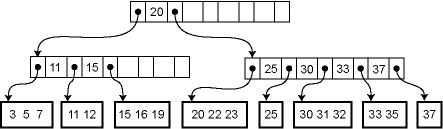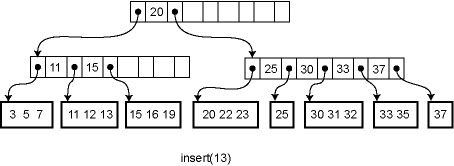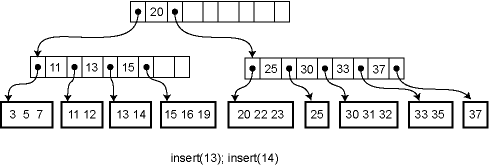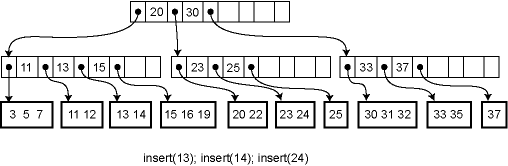B-Trees

The idea we saw earlier of putting multiple set (list, hash table) elements together into large chunks that exploit locality can also be applied to trees. Binary search trees are not good for locality because a given node of the binary tree probably occupies only a fraction of any cache line. B-trees are a way to get better locality by putting multiple elements into each tree node.

B-trees were originally invented for storing data structures on disk, where locality is even more crucial than with memory. Accessing a disk location takes about 5ms = 5,000,000ns. Therefore, if you are storing a tree on disk, you want to make sure that a given disk read is as effective as possible. B-trees have a high branching factor, much larger than 2, which ensures that few disk reads are needed to navigate to the place where data is stored. B-trees may also useful for in-memory data structures because these days main memory is almost as slow relative to the processor as disk drives were to main memory when B-trees were first introduced!

A B-tree of order m is a search tree in which each nonleaf node has up to m children. The actual elements of the collection are stored in the leaves of the tree, and the nonleaf nodes contain only keys. Each leaf stores some number of elements; the maximum number may be greater or (typically) less than m. The data structure satisfies several invariants:

1. Every path from the root to a leaf has the same length
2. If a node has n children, it contains n−1 keys.
3. Every node (except the root) is at least half full
4. The elements stored in a given subtree all have keys that are between the keys in the parent node on either side of the subtree pointer. (This generalizes the BST invariant.)
5. The root has at least two children if it is not a leaf.

For example, the following is an order-5 B-tree (m=5) where the leaves have enough space to store up to 3 data records:Because the height of the tree is uniformly the same and every node is at least half full, we are guaranteed that the asymptotic performance is O(lg n) where n is the size of the collection. The real win is in the constant factors, of course. We can choose m so that the pointers to the m children plus the m−1 elements fill out a cache line at the highest level of the memory hierarchy where we can expect to get cache hits. For example, if we are accessing a large disk database then our "cache lines" are memory blocks of the size that is read from disk.

Lookup in a B-tree is straightforward. Given a node to start from, we use a simple linear or binary search to find whether the desired element is in the node, or if not, which child pointer to follow from the current node.

Insertion and deletion from a B-tree are more complicated; in fact, they are notoriously difficult to implement correctly. For insertion, we first find the appropriate leaf node into which the inserted element falls (assuming it is not already in the tree). If there is already room in the node, the new element can be inserted simply. Otherwise the current leaf is already full and must be split into two leaves, one of which acquires the new element. The parent is then updated to contain a new key and child pointer. If the parent is already full, the process ripples upwards, eventually possibly reaching the root. If the root is split into two, then a new root is created with just two children, increasing the height of the tree by one.

For example, here is the effect of a series of insertions. The first insertion (13) merely affects a leaf. The second insertion (14) overflows the leaf and adds a key to an internal node. The third insertion propagates all the way to the root.Deletion works in the opposite way: the element is removed from the leaf. If the leaf becomes empty, a key is removed from the parent node. If that breaks invariant 3, the keys of the parent node and its immediate right (or left) sibling are reapportioned among them so that invariant 3 is satisfied. If this is not possible, the parent node can be combined with that sibling, removing a key another level up in the tree and possible causing a ripple all the way to the root. If the root has just two children, and they are combined, then the root is deleted and the new combined node becomes the root of the tree, reducing the height of the tree by one.

Further reading: Aho, Hopcroft, and Ullman, Data Structures and Algorithms, Chapter 11.